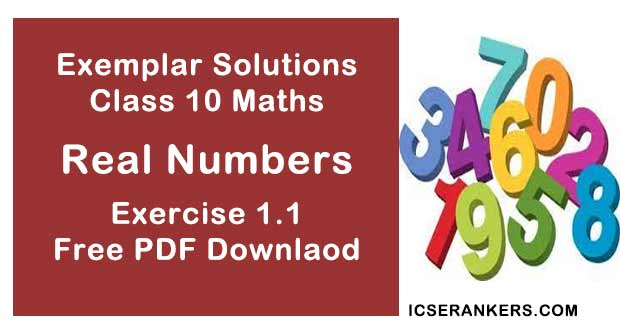# Chapter 1 Real Number NCERT Exemplar Solutions Exercise 1.1 Class 10 MathsChapter Name NCERT Maths Exemplar Solutions for Chapter 1 Real Numbers Exercise 1.1 Book Name NCERT Exemplar for Class 10 Maths Other Exercises Exercise 1.2Exercise 1.3Exercise 1.4 Related Study NCERT Solutions for Class 10 Maths

### Exercise 1.1 Solutions

1. For some integer m, every even integer is of the form:

(A) m

(B) m +1

(C) 2m

(D) 2m+1

Solutions

(C) 2m
Integers which are divisible by 2 are called even integers.
So, every even integer must be a multiple of 2. So, we can conclude that, for any integer m,
Where, m = 1, 2, 3, 4....n, even integer is in the form of 2 × m = 2m.

2. For some integer q, every odd integer is of the form

(A) q

(B) q + 1

(C) 2q

(D) 2q + 1

Solutions

(D) 2q+1
Integers which are not divisible by 2 are called odd integers.
And integer which is a multiple of 2 is an even integer, if 1 is added to any integer which is multiplied by 2, it gives an odd integer.
So, we can say that, for any integer ‘q’, every odd integer is of the form 2q+1.

3. n2 – 1 is divisible by 8, if n is
(A) an integer
(B) a natural number
(C) an odd integer
(D) an even integer

Solutions

(C) an odd integer
Let a = n2 – 1
In the above equation, n can be even or n can be odd.
Let us say that n is even integer.
So, If n = even i.e., n = 2x, where x is an integer,
We get,
a = (2x)2 - 1
⇒ a = 4x2 - 1
Let , x = 0,
a = 4(0)2 - 1
= 0 - 1 = -1
⇒ a = -1 is not divisible by 8.
Let us say that n = odd.
When n = odd i.e.
n = 2x + 1, where x is an integer,
We have,
a = (2x + 1)2 - 1
⇒ a = 4x2 + 4x + 1 - 1
⇒ a = 4x2 + 4x
⇒ a = 4x(x + 1)
At x = 0,
a = 4(0)(0 + 1)
⇒ a = 0 which is divisible by 8.
So, we can conclude that, n2 - 1 is divisible by 8 when n is an odd integer.

4. If the HCF of 65 and 117 is expressible in the form 65m – 117, then the value of m is

(A) 4

(B) 2

(C) 1

(D) 3

Solutions

(B) 2
If we find the HCF of 65 and 117, we get,
117 = 1×65 + 52
65 = 1× 52 + 13
52 = 4 ×13 + 0
The HCF of 65 and 117 is 13.
Putting value in the question, we get
65m – 117 = 13
⇒ 65m = 117+13
⇒ 65m = 130
∴ m = 130/65
⇒ m = 2

5. The largest number which divides 70 and 125, leaving remainders 5 and 8, respectively, is

(A) 13

(B) 65

(C) 875

(D) 1750

Solutions

(A) 13
We have to find the largest number which divides 70 and 125, leaving remainders 5 and 8.
So, we can write it as,
The largest number which divides (70 – 5), and (125 – 8), as 5 and 8 are remainder,
Ultimately we have to find the Highest Common Factor of 65 and 117
On solving,
Multiples of 65 = 1, 5, 13, 65
Multiples of 117 = 1, 3, 9, 13, 39, 117
Common multiple = 1, 13
So, HCF= 13
Therefore, 13 is the number which divides 70 and 125, leaving remainders 5 and 8.

6. If two positive integers a and b are written as a = x3y2 and b = xy3 ; x, y are prime numbers,, then HCF (a, b) is

(A) xy

(B) xy2

(C) x3y3

(D) x2y2

Solutions

(B) xy2
To find the HCF of a and b, we have to see the common terms in a and b, on solving, we get,
HCF = xy2

7. If two positive integers p and q can be expressed as p = ab2 and q = a3b; a, being  prime numbers, then LCM (p q) is:

(A) ab

(B) a2b2

(C) a3b2

(D) a3b3

Solutions

(C) a3b2
p = ab2
q = a3b
To find LCM we see the highest powers of each number.
So, LCM  = a3b3

8. The product of a non-zero rational and an irrational number is
(A) always irrational
(B) always rational
(C) rational or irrational
(D) one

Solutions

(A) always irrational
Product of a non-zero rational and an irrational number is irrational.

9. The least number that is divisible by all the numbers from 1 to 10 (both inclusive) is

(A) 10

(B) 100

(C) 504

(D) 2520

Solutions

(D) 2520
We have to find the LCM of numbers from 1 to 10.
We will first see the multiples of numbers from 1 – 10,
1 = 1, 2 = 2, 3 = 3
4 = 2 × 2, 5 = 5, 6 = 2 × 3 , 7 = 7
8 = 2 × 2 × 2, 9 = 3 × 3, 10 = 2 × 5
LCM of all numbers 1 to 10 = 1 × 2 × 3 × 2 × 5 × 7 × 2 × 3 = 2520

10. The decimal expansion of the rational number 14587/1250 will terminate after:
(A) One decimal place
(B) two decimal places
(C) Three decimal places
(D) four decimal places

Solutions
(D) Four decimal places
On dividing we get,
14587/1250 = 11.6696